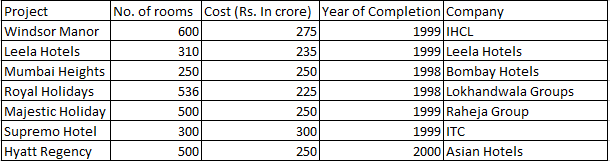# Cumulative DI for CAT

0
2922

We are providing the most important questions for Cumulative DI for CAT:5 very useful questions for CAT cumulative concepts and di and LRDI puzzle questions and solutions tricks for CAT

Cumulative DI for CAT:

Download the CAT Verbal Ability PDFs/Ebooks and all Quantitative aptitude questions PDF.

Instructions:

The following table gives the number of rooms in different hotels in Mumbai and the cost of building each one of them. Note that all the projects started in 1997Note: All projects start in 1997.

Question 1:

Which of the following had the least cost per room?

a) Lokhandwala Group
b) Raheja Group
c) IHCL
d) ITC

Question 2:

Which of the following has the maximum number of rooms per crore of rupees?

a) IHCL
b) Raheja Group
c) Lokhandwala Group
d) ITC

Question 3:

Assume that the cost of the project is incurred in the year of completion; interest is charged at the rate of 10% per annum. What is the cost incurred for projects completed in 1998?

a) Rs. 475 crore
b) Rs. 500 crore
c) Rs. 522.5 crore
d) Rs. 502.5 crore

Question 4:

Assume that the cost of the project is incurred in the year of completion; interest is charged at the rate of 10% per annum. What is the cost incurred for projects completed in 1999?

a) Rs. 1,282.6 crore
b) Rs. 1,270 crore
c) Rs. 1,805.1 crore
d) Rs. 1,535 crore

Question 5:

What is the approximate cost incurred for projects completed by 2000?

a) Rs. 1,785
b) Rs. 2,140
c) Rs. 2,320
d) None of these

Answers and Solutions for Cumulative DI for CAT:

Solutions:

The cost per room equals (total cost)/(total rooms)
To calculate the least cost per room, we have to only check IHCL and Lokhandwala Group. A simple inspection will remove all other companies as they have more cost and lesser rooms than either of the two.
Cost per room of IHCL = 275/600 = 45.83 lakhs
Cost per room of Lokhandwala = 225/536 = 41.97 lakhs.
Hence, the cost per room is least for Lokhandwala Group.

For the above answer, the ratio of number of rooms to the cost should be maximum. It is maximum for lokhandwala group which is 536/225 = 2.38

Total cost in 1997 = 250 + 225 = 475 Crores.
These projects were completed in 1998 => Interest for 1 year must be added to the cost.
Interest = $$\frac{475*1*10}{100}$$ = 47.5 Crores
Total Amount = 475 + 47.5 = 522.5 Crores.

Interest for 2 years must also be added to this cost => $$1060(1+(0.1))^2$$ = 1060 * 1.21 = Rs 1282.6 Crores